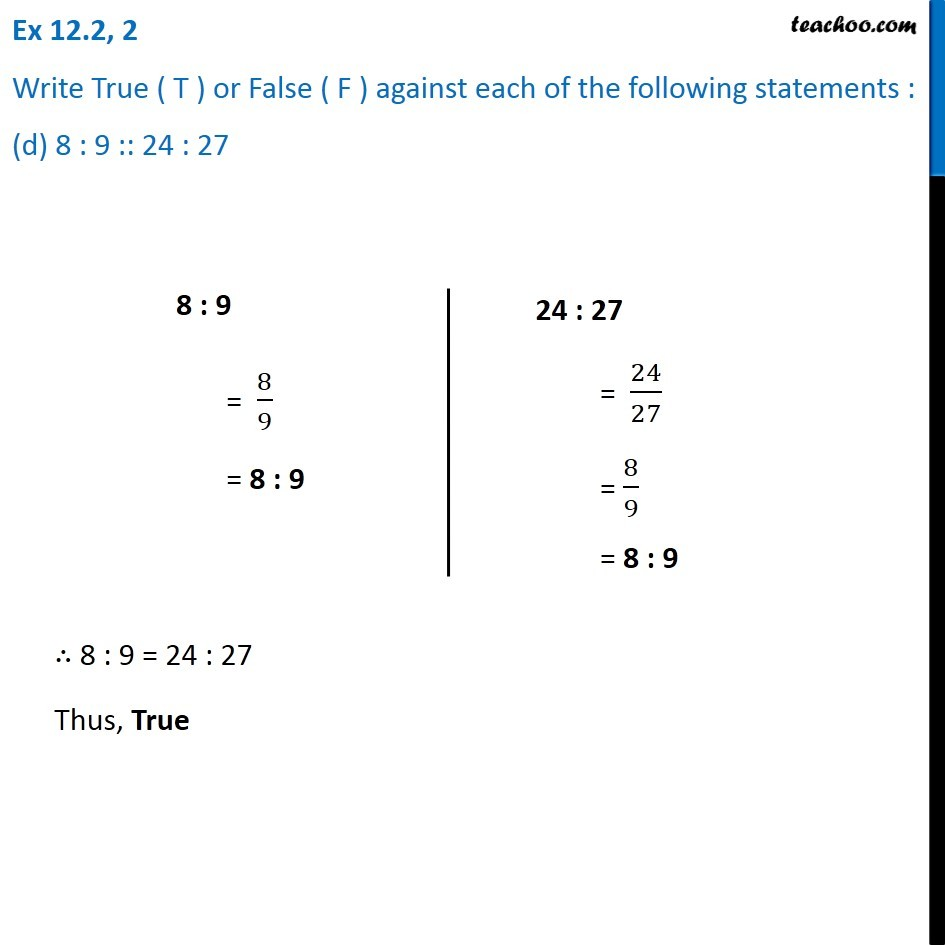Ex 12.2

Chapter 12 Class 6 Ratio and Proportion
Serial order wiseLearn in your speed, with individual attention - Teachoo Maths 1-on-1 Class

### Transcript

Ex 12.2, 2 Write True ( T ) or False ( F ) against each of the following statements : (d) 8 : 9 :: 24 : 278 : 9 = 8/9 = 8 : 9 24 : 27 = 24/27 = 8/9 = 8 : 9 ∴ 8 : 9 = 24 : 27 Thus, True